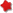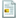Chaos Cluster has returned.

# Chaos Cluster

###Author Topic: Intro to Tinywarz Part: 4 Leveling your Commander  (Read 2636 times)

#### Lomaz

• Experienced Member
•• Posts: 191
• Karma: +0/-0##### Intro to Tinywarz Part: 4 Leveling your Commander
« on: November 26, 2010, 05:24:09 am »
Alright, this seems to be a big issue i find, if you were to graph everyone's level on Dropshock, i believe there'd be a huge lull (not LOL) between levels 5 and 25, it seems people either decide leveling is too hard and bandits are too scarce (because they don't know the proper ways for looking for them) or they figure it out and have no trouble at all leveling to at least the low thirty's.

One main thing people do wrong when they first start, if their intent is to level their commander, is drop several units, Unless a crew has E/E, and 3 Merits, the commander WILL ONLY RECEIVE 10% EXP FROM ANYTHING THAT UNIT KILLS, and none if the unit is 25+ from commander, and bonuses from exp mods (CAS/CAM) do not transfer over.  Thus the best way to level, is to make a single unit, with decent guns/armor, and go "solo"

So, to locate bandits, the way frizz coded each planet it simple, each planet is set up in sets of 30x30 quadrants, in the middle 10x10 sector is where Bandits will re-spawn if there is NOTHING in the quadrant, so each planet looks something like this:

Let X represent an empty sector, and Y represent where bandits may spawn if all touching sectors are clear of any bandits/units/buildings/minerals

X X X X X X X X X X X X
X Y X X Y X X Y X X Y X
X X X X X X X X X X X X
X X X X X X X X X X X X
X Y X X Y X X Y X X Y X
X X X X X X X X X X X X
X X X X X X X X X X X X
X Y X X Y X X Y X X Y X
X X X X X X X X X X X X

So to optimize your spawns, you want to run your commander along a line like this:

X X X X X X X X X X X X
X Y X X Y X X Y X X Y X
X X X X X X X X X X X X
------------------------
X X X X X X X X X X X X
X Y X X Y X X Y X X Y X
X X X X X X X X X X X X

Hopping up and down either side of the red line so both the top and bottom spawns can see you and come after you. Also, if on a higher spawn planet like Konu, you may find it worthwhile to stay at a corner of 4 spawns like this:

X X X | X X X
X Y X | X Y X
X X X | X X X
------|------
X X X | X X X
X Y X | X Y X
X X X | X X X

Hopping around the corners until bandit bases/minerals spawn (as soon as all bandits in a quad are dead, if no minerals are there, or buildings, more bandits will spawn)

I have gone nearly half a cycle doing this when I'm lucky, and had a stack of 50+ bandits all around me when i was finished, it's i find, the quickest way to level if you're strong enough to kill quickly.

Another quick easy way to boost exp is with a CAS/CAM, 2 mods that do 50% and 25% exp respectively, off each kill made by unit it's equipped on.

Roughly the same tactic can be used in a Dragbase, Dragbases need a bit more command, but buildings give your units bonuses you wouldn't otherwise get, to help you killing, when you do this on Konu, build a base (guide on buildings coming soon), and drop it on Konu, if you look at the first map shown, Your base will replace one Y, so after dropping you'll be at this: (assuming you don't get a wall drop)

B = Base drop location

X X X X X X X X X
X Y X X Y X X Y X
X X X X X X X X X
X X X X X X X X X
X Y X X B X X Y X
X X X X X X X X X
X X X X X X X X X
X Y X X Y X X Y X
X X X X X X X X X

So you can then use drones/probes/simple units to drag bandits from all 8 surrounding spawn points to your base where your commander waits to kill them. i personally don't do this, but it's quite effective.